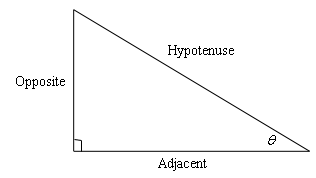# "Soh Cah Toa"

Author:
Kenneth
Maybe you've heard of the expression "Soh Cah Toa" before. It turns out that that expression relates to Trigonometry!Look at the right triangle above. You might be wondering what the weird sign "" is. (theta, pronounced "they-ta") is a Greek symbol that is commonly used as a trigonometric variable for the measure of an angle.
If we relate using , , and , we have three new formulas to remember, although they're not too bad.
To help you memorize these three formulas, mathematicians have created a catchy phrase: "soh cah toa." It simply states that "sin is opposite over hypotenuse," "cosine is adjacent over hypotenuse," and "tangent is opposite over adjacent." Take some time to memorize this phrase.Remember that the sides change names when you change the angle. However, the trigonometric formulas stay the same!

## Review

How do you find the sine of an angle?

How do you find the cosine of an angle?

How do you find the tangent of an angle?